Function Repository Resource:

# RealEuclideanDistance

Get the Euclidean distance between two real vectors without using absolute value

Contributed by: Ed Pegg Jr
 ResourceFunction["RealEuclideanDistance"][u,v] gives the Euclidean distance between real vectors u and v without using Abs.

## Examples

### Basic Examples (3)

Compute the real Euclidean distance between two points:

 In:=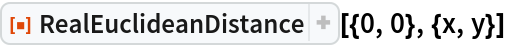Out=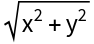Compute in three dimensions:

 In:=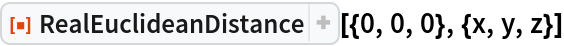Out=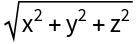Using numeric values:

 In:=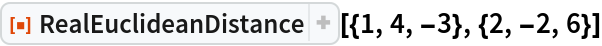Out=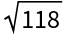### Properties and Relations (2)

EuclideanDistance adds Abs for variables and complicated expressions, making them more complicated. Often, that extra complication will make Simplify more difficult:

 In:=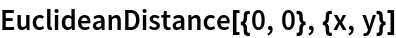Out=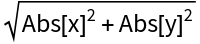Compare with RealEuclideanDistance:

 In:=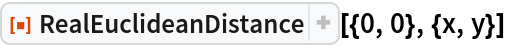Out=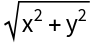## Requirements

Wolfram Language 11.3 (March 2018) or above

## Version History

• 1.0.1 – 29 January 2021
• 1.0.0 – 05 February 2019